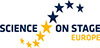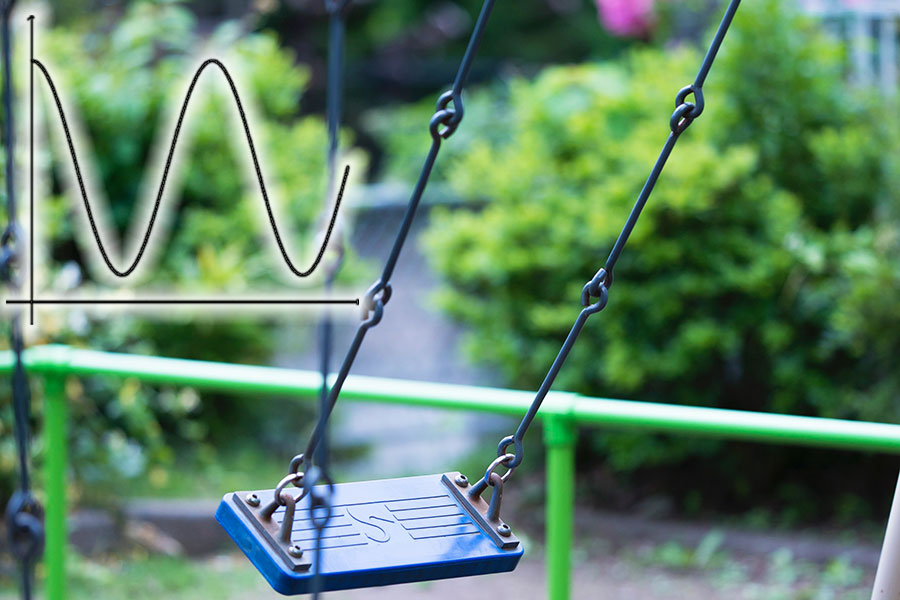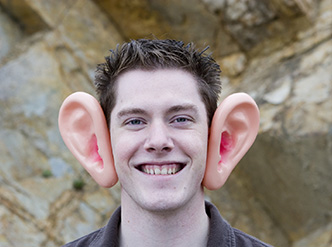# Dance, tangles, and topology! Teach article

This activity was presented at the Science on Stage Festival 2022Everybody dance now: students hold ropes and dance to form a topological tangle. Using fraction arithmetic, the knot will finally be untied!

## Introduction

Topology is often excluded from educational programs due to its complex formalism, but, at basic levels, it is a rich, interesting topic that is very suitable for games: false and true knots, the cutting of Möbius strips, folded papers, holes, puzzle games, and magic tricks are all examples. Here, the concept of Conway’s rational tangles is used to propose a ‘dance’ based on simple movements of four people holding ropes.  While they’re swapping their positions, a tangle forms between their ropes. To untie the knot, it is necessary to assign rational numbers to knots and operations to each dance step. Using only fraction arithmetic, the knots will finally be untied!

In secondary schools, it could be proposed as a team competition to practise arithmetic, while, in primary schools, it could be used without the more complex mathematics as a precoding activity.

## Goals and objectives

The mathematical field of topology turns out to be rather intuitive and stimulating at a playful level, mainly with the aid of commonly used objects, such as ropes, scarfs, earphone cables, and doughnuts.

The general aim of this activity is to introduce simple elements of topology and open the mind to a vision of forms different from those proposed by geometry or algebra. Topology deals with connections between points of an object, to give mathematical descriptions based to the presence of ‘holes’, ‘continuity’, faces and surfaces, nodes and overlaps, the concepts of internal and external, and borders. Topology is a complex mathematical field, but these intuitive concepts can also be used to give a sort of playful ‘order’ to objects and affinities between different objects, through continuous transformations, such as knots, tangles, and cutting with scissors.

## How can teachers implement the project in their classrooms?

These activities can be carried out in one or two lessons, depending on how much time is taken for discussion and recap of mathematical topics. They are described at a suitable level for students aged 11–16; however, they can be adapted for students aged 17–19 too. They can be used to simply introduce the topology environment; to review/consolidate the teaching of fractions and simple arithmetic; or as a starting point to go deeper into the topic, finding rules and connections between knots and algebra. More detail on how to adapt the activity for different age groups and incorporate it into the curriculum can be found in the supporting material.

## Activity 1: Introduction and quick roundup of topological games

Firstly, we introduce some simple and intuitive topological concepts using simple games and tricks. Additionally, if teachers would like to present some background to topology, simple video introductions to topology and knots can be found in the Resources section.

#### Materials

• Some simple tricks based on ropes and false knots (see the Resources section for suggestions)
• For tricks and games: strings, scarf, rings, and bottle corks, depending on which tricks are selected
• A collection of common objects with different topologies, like clothing, pasta shapes, bottles, and pretzels
• Paper
• Scissors
• Pencils
• Sticky tape or glue

#### Procedure

1. Firstly, the teacher can show a couple of tricks based on ropes and false knots, such as the scarf that is not worn or take the ring out of the ropes. These can be demonstrated (perhaps with the teacher asking for suggestions or explanations from the class).
2. If you have more time, a couple of students can be invited to the front to try to solve a rope handcuff puzzle: each of them is handcuffed by a rope woven through their partner’s rope, and together they must find a way to untie themselves without cutting or unthreading the rope from their wrists.
3. Introduce the topological idea of holes by asking the class how many holes there are in common objects. If students get the answer wrong, this is an opportunity to clarify the topological definition of holes and introduce some vocabulary. For example, a sock is thought to have one hole, whereas topologically speaking, it has no hole at all, because it is homoeomorphic to a piece of fabric.
4. A nice example to demonstrate this principle is to show how a coffee cup is morphologically equivalent to a donut. Can students think of any other objects that are homoeomorphic?
5. Have students create a Möbius strip from paper and tape. Ask them how many surfaces it has and encourage them to check by drawing a dot and then drawing a line along the strip until they reach the dot again.
1. Ask students cut the Möbius strip along the middle line to produce intertwined, elongated, or twisted rings.

## Activity 2: The topology dance

In this activity, pairs of students hold onto either end of pieces of rope and then ‘dance’ specific movements to create knots. They then learn how these dance moves can be assigned mathematical functions, thus allowing the knot and its solution (untying) to be described mathematically.

### Tip for the teacher

Try the dance sequences on your own with two strings and four weights tied to the ends!

#### Materials

• Open area of at least 3×6 m2 for dancing
• Some means of marking squares on the ground, e.g., tape (inside) or chalk (outside)
• Pieces of rope 2 m long, one for every two students
• The movement figures for rotations and swaps (ideally printed to show the students)
• Pens and paper (for writing sequences)
• Some music to play during the dance; folk music works especially well
##### Part 1: Introduction to the topology dance
1. Mark out squares on the ground.
2. Split the students into groups of four.
3. Call up four students to demonstrate in front of the class, and place them at the corners of the square.
4. Run through the rotation (R) step and the swap (S) step. Note that the rotation must be performed in one direction only (e.g., clockwise) and that during the swap rope A must cross over rope B. It could be useful to show the steps and figures on a screen to the whole class.
1. Ensure that all students understand the movements.
2. Then have the groups practice in their own squares.
##### Part 2: Warm-up dance, goal, and rules of the game
1. Choose a simple dance sequence (e.g., SSRSSRSSS), turn on the music, and guide your students step by step through the dance.
2. At the end, stop the music: a knot has formed between their ropes.
3. Now the goal is to untie it, using the same movements as before (it is forbidden to do the movements in reverse!).
4. The teacher should now show an example of a solution (e.g., RSRSSRSSSS), and how it works to untie the tangle.
5. Stop the dance and discuss how you would find a solution. It is now that topological algebra helps us. Show your students that, incredibly, each step corresponds to an operation and each knot to a rational number. It could be useful to recap fraction rules now, if needed.

### Scheme for the mathematical operations

• Ropes untied (initial position) equals 0
• Doing the swap corresponds to the operation +1, that is, adding 1 to the previous number
(e.g., 2 + 1 =3)
• Doing a rotation corresponds to the negative reciprocal, that is inverting and changing the sign of the previous number (e.g., the negative reciprocal of 3 is −⅓)

The knot obtained thus corresponds to a rational number (in the previous example, it corresponds to 7/3). Now students have to search for a sequence of operations that will eventually lead back to zero.

1. Optional: point out how an abstract mathematical algorithm can be used to solve problems with strings and tangles to show students the transversal power of mathematics. Point out that, using this approach, every possible kind of knot corresponds to a rational number and that maths can describe it (although not so easily). Some simple videos on the science of knots can be found in the Resources section.
##### Part 3: Group dances
1. Choose other algorithms (and music) to dance to and give the students the sequence on paper.
2. Have the students work through the mathematical operations to calculate the rational number for their knot.
3. Older students can work out how to solve (untie) the knot with only the allowed moves using equations.
For a simplified version, the students can instead be given different sequences of commands as potential solutions and be asked to calculate which will lead to untangling of the ropes. For this, it is necessary to calculate the relative fractions up to the final result.
1. Start the dance and have the teacher call out the dance moves. Pay attention not to make mistakes. An error could cause chaos later! Students should practise.
2. Stop the music and check all students have the correct knot.
3. Now the students should test their solution sequence.

A nice variation is a team competition: the teacher gives the initial steps, and the students have to decide on the best algorithm to untie the knots. When the music is turned on again after the knots are checked, the first team to untie the knot wins.

Considering that there is no single solution algorithm, most teams should be able to untie the knot, but there is still a shortest algorithm, so the fastest team could win. Let students compete on the aspect of optimization of the procedure, which is a more purely computational skill.

##### Optional extensions
• Have students propose and then demonstrate extreme dances (very tangled).
• Introduce infinity. An infinitive emerges if you invert zero (one rotation from the initial position). You can explore this by adding one to the infinitive (that is, doing a swap) and observing that the pattern does not change.
• Enclose the initial knot in a plastic bag then go on with the dance. An antiknot is formed outside of the bag. Breaking the plastic bag will show that the two knots sum to zero.

## Conclusion

Overall, the activities can be used more generally to stimulate problem solving and to demonstrate how mathematics can be used to describe real-world phenomena. However, they can also be used to explore more specific curriculum topics in an engaging context, such as computational thinking, operations with fractions (sum, inverse, opposite sign), computational thinking, dimensionality (e.g., lines versus surfaces), nodes, rational numbers, solving equations, and algorithms.

### References

 Goldman JR, Kauffman LH (1997) Rational tangles. Advances in Applied Mathematics, 18: 300–332. doi: 1.1006/aama.1996.0511

 Davis T (2017) Conway rational tangles: http://www.scienceinschool.org/wp-content/uploads/2023/05/Tom-Davis-tangles.pdf

### Author(s)

Stefano Alberghi is a secondary school physics and maths teacher. He collaborates with the Science Gym in Faenza (Italy). He’s been a science trainer of teachers in mathematical machines, dealt with physics and maths projects in amusement parks, developed Matebilandia (maths activities in Mirabilandia park, Italy), and written a book about this. Previously, he was a climate researcher.

CC-BY
Text released under the Creative Commons CC-BY license. Images and supporting materials: please see individual descriptions.

## Related articles

Teach### Teaching binary code with a secret word challenge

Do you find the binary system complicated? With this activity, your students will find it as easy as…

Teach### Graphing stories

Sketch graphs from ‘story’ videos of everyday events to help students understand the basic features of graphs and how to interpret…

Understand### Damn lies

Do you have more than the average number of ears? Is your salary lower than average? When will the next bus arrive? Ben Parker attempts to convince…